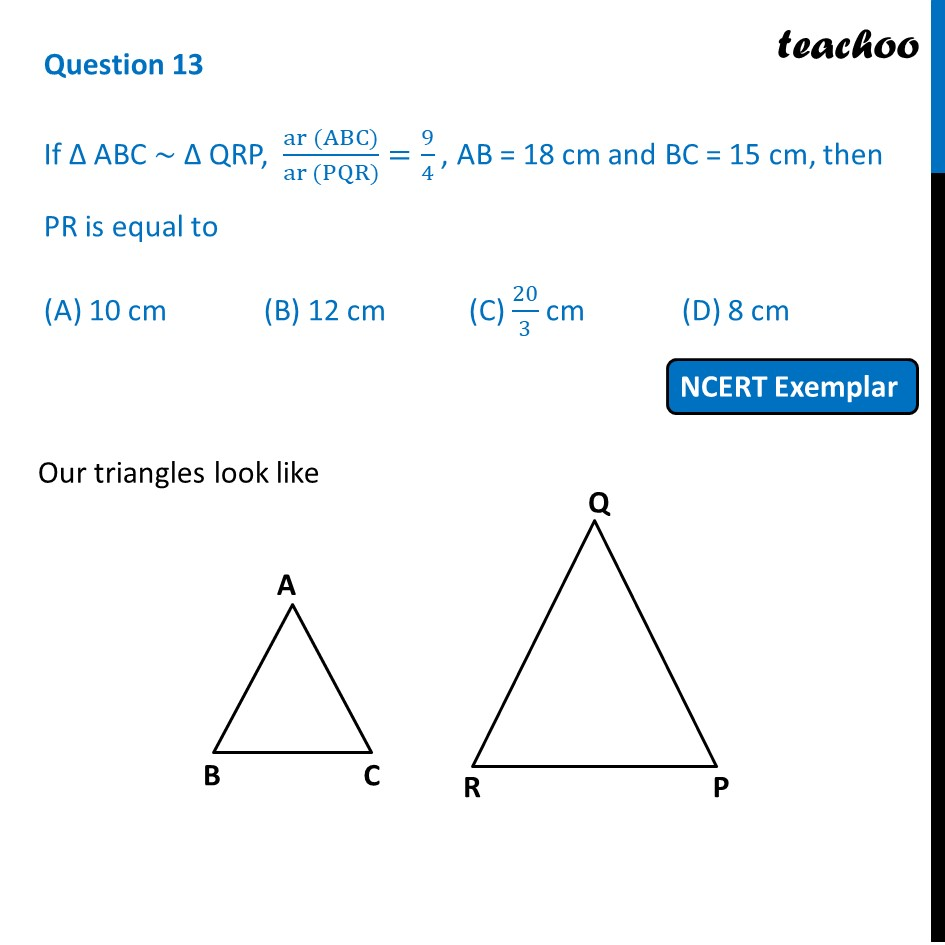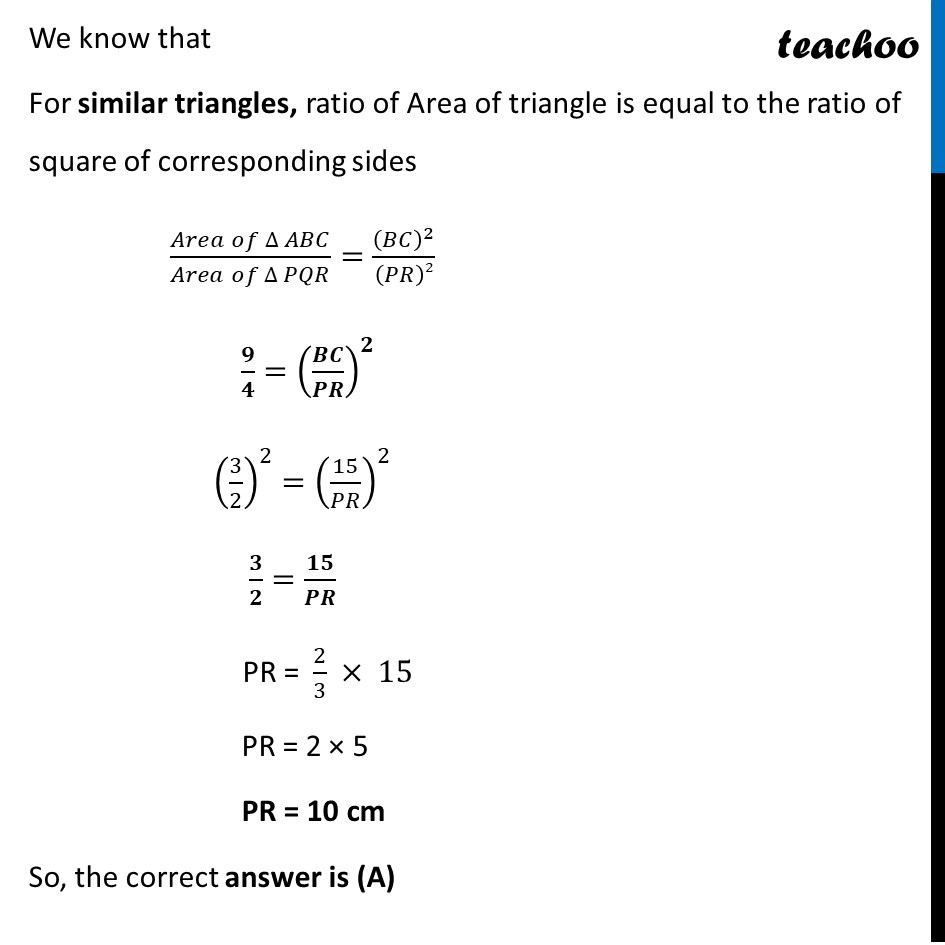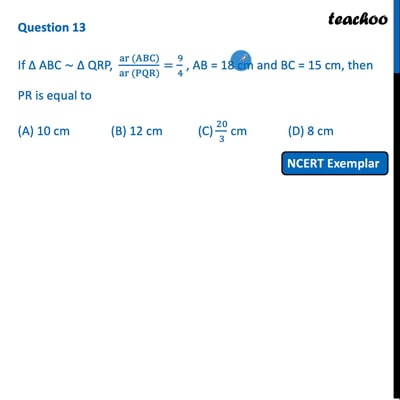NCERT Exemplar - MCQ

Chapter 6 Class 10 Triangles
Serial order wise

## (D) 8 cmThis video is only available for Teachoo black users

Introducing your new favourite teacher - Teachoo Black, at only ₹83 per month

### Transcript

Question 13 If ∆ ABC ~ ∆ QRP, (ar (ABC))/(ar (PQR))=9/4 , AB = 18 cm and BC = 15 cm, then PR is equal to (A) 10 cm (B) 12 cm (C) 20/3 cm (D) 8 cm Our triangles look like We know that For similar triangles, ratio of Area of triangle is equal to the ratio of square of corresponding sides (𝐴𝑟𝑒𝑎 𝑜𝑓 ∆ 𝐴𝐵𝐶)/(𝐴𝑟𝑒𝑎 𝑜𝑓 ∆ 𝑃𝑄𝑅)=(𝐵𝐶)^2/(𝑃𝑅)2 𝟗/𝟒=(𝑩𝑪/𝑷𝑹)^𝟐 (3/2)^2=(15/𝑃𝑅)^2 𝟑/𝟐=𝟏𝟓/𝑷𝑹 PR = 2/3 × 15 PR = 2 × 5 PR = 10 cm So, the correct answer is (A)